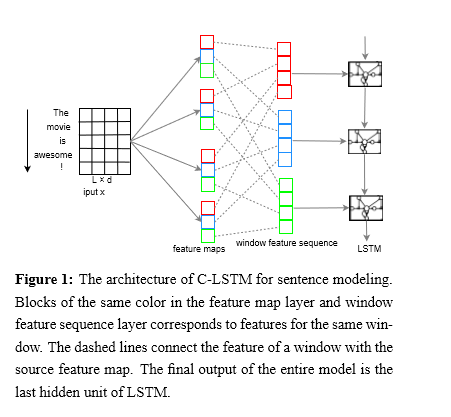## 中文短文本分类实例九-CRNN（A C-LSTM Neural Network for Text Classiﬁcation）_a c-lstm neural network for text classification_Macropodus的博客-程序员宅基地

CRNN（A C-LSTM Neural Network for Text Classiﬁcation），是Chunting Zhou等提出的一种联合CNN和RNN的优点的混合神经网络，可称之为"卷积-递归"神经网络。

不同于RCNN先对文本句子上下文本信息、以及word-embedding本身特征的提取，CRNN中的CNN可以看成另外一种形式的句子信息的Embedding（char、word and n-gram），它具有实际意义上的解释性；也可以看成是对局部短语特征的提取，用于文本表达和文本分类。而LSTM便很善于捕捉文本的全局特征，在它接入CNN后，可以有效缓解CNN对于长文本句子信息捕获不足的缺点。

其实现在看来，CRN算是比较古老的版本的了，也没什么特别多可说的，不就是把CNN和RNN堆砌起来么，没什么那么多值得大惊小怪的。

github项目地址:

2.1  CRNN模型图2.2  tricks等

初始化: 词向量dim=100, OOV词=随机初始化为均匀分布(uniform distribution [-0.25, 0.25])

规范化(Regularization): dropout 和 L2

似乎就没有了

3.1  　很简单的一个模型，就是把CNN和LSTM连在一起，似乎就好了

github项目地址:

3.２　核心代码

``````    def create_model(self, hyper_parameters):
"""
构建神经网络
:param hyper_parameters:json,  hyper parameters of network
:return: tensor, moedl
"""
super().create_model(hyper_parameters)
x = self.word_embedding.output
embedding_output_spatial = SpatialDropout1D(self.dropout_spatial)(x)

if self.rnn_units=="LSTM":
layer_cell = LSTM
elif self.rnn_units=="GRU":
layer_cell = GRU
elif self.rnn_units=="CuDNNLSTM":
layer_cell = CuDNNLSTM
elif self.rnn_units=="CuDNNGRU":
layer_cell = CuDNNGRU
else:
layer_cell = GRU
# CNN
convs = []
for kernel_size in self.filters:
conv = Conv1D(self.filters_num,
kernel_size=kernel_size,
strides=1,
kernel_regularizer=regularizers.l2(self.l2),
bias_regularizer=regularizers.l2(self.l2),
)(embedding_output_spatial)
convs.append(conv)
x = Concatenate(axis=1)(convs)
# Bi-LSTM, 论文中使用的是LSTM
x = Bidirectional(layer_cell(units=self.rnn_units,
return_sequences=True,
activation='relu',
kernel_regularizer=regularizers.l2(self.l2),
recurrent_regularizer=regularizers.l2(self.l2)
))(x)
x = Dropout(self.dropout)(x)
x = Flatten()(x)
# 最后就是softmax
dense_layer = Dense(self.label, activation=self.activate_classify)(x)
output = [dense_layer]
self.model = Model(self.word_embedding.input, output)
self.model.summary(120)``````

### sql 右键编辑200行查询很慢_sql server 2014 右键 很慢-程序员宅基地

sql右键编辑200速度很慢解决办法！_sql server 2014 右键 很慢

### 通过 pom 设置 Maven 通过 JDK 1.8 进行编译 ——maven-compiler-plugin插件-程序员宅基地

maven项目会用maven-compiler-plugin默认的jdk版本来进行j编译，如果不指明版本就容易出现版本不匹配的问题，可能导致编译不通过的问题。解决办法：在pom文件中配置maven-compiler-plugin插件（以jdk1.8）。1、方式一<properties> <maven.compiler.target>1.8</maven.compiler.target> <maven.compiler.source

### C#操作内存_c# 写内存-程序员宅基地

using System;using System.Text;using System.Diagnostics;using System.Runtime.InteropServices;namespace PlantsVsZombiesTool{ publicabstractclass Helper { [DllImpo_c# 写内存

### 毕设题目：Matlab手写或印刷数字字母识别_基于matlab的手写数字识别毕设_海神之光的博客-程序员宅基地

1 案例背景4 现成案例（代码+参考文献）1【手写数字识别】基于matlab CNN网络手写数字识别分类【含Matlab源码 1286期】2【手写数字识别】基于matlab Fisher分类手写数字识别【含Matlab源码 505期】3【手写数字识别】基于matlab GUI BP神经网络手写数字识别【含Matlab源码 1118期】4【手写数字识别】基于matlab GUI RBM神经网络手写数字识别【含Matlab源码 1109期】5【手写数字识别】基于matlab GUI SVM手写数字识_基于matlab的手写数字识别毕设